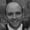FREELessons: 30Length: 4.8 hours• Overview
• Transcript

# 5.5 Demo: FizzBuzz

In this demo application, we will be solving a problem that is often posed in basic development job interviews. It’s known as the "FizzBuzz problem". The basic idea is that you need to go through each number in a given range and for each one output "Fizz" if it's divisible by 3 or "Buzz" if it's divisible by 5. If the number is divisible by both 3 and 5, output "FizzBuzz”, and if none of the conditions are true, just output the number. Let's have a go!